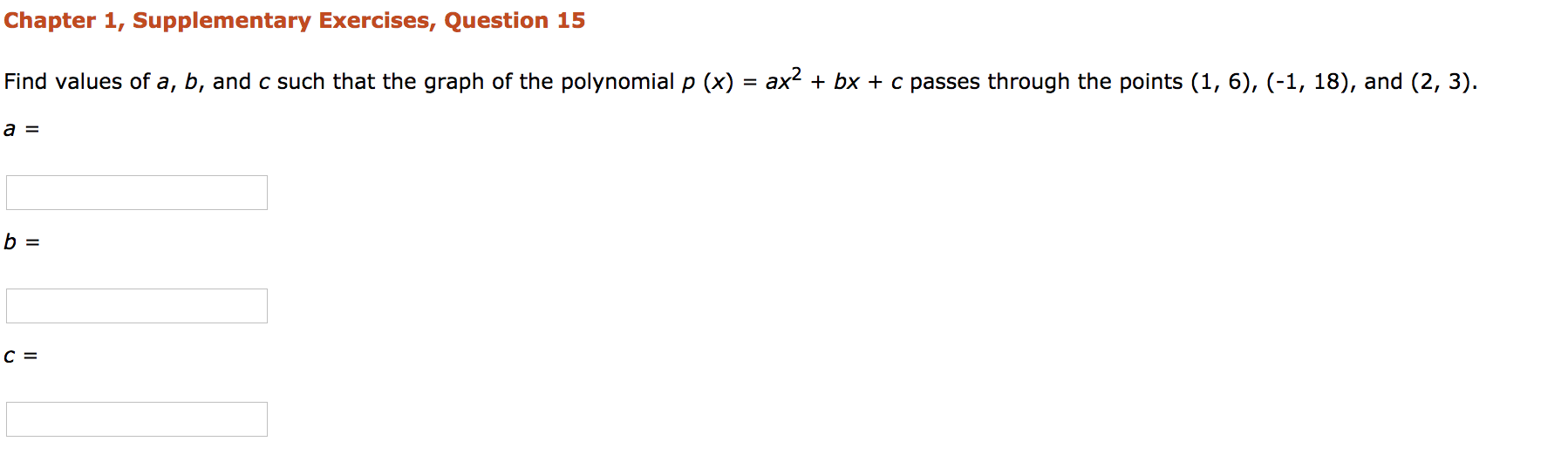Home / Answered Questions / Other / chapter-1-supplementary-exercises-question-15-find-values-of-a-b-and-c-such-that-the-graph-of-the-po-aw670

# (Solved): Chapter 1, Supplementary Exercises, Question 15 Find Values Of A, B, And C Such That The Graph Of Th...Chapter 1, Supplementary Exercises, Question 15 Find values of a, b, and c such that the graph of the polynomial p (x) = ax2 + bx + c passes through the points (1, 6), (-1, 18), and (2, 3). a = b =

We have an Answer from Expert